WHCSRL 技术网

# 【C++】进制转换

11%%2=1   11/2=5   5%%2=1   5/2=2
2%%2=0    2/2=1    1%%2=1   1/2=0

## 1.什么是进制

### 十进制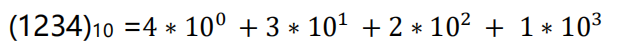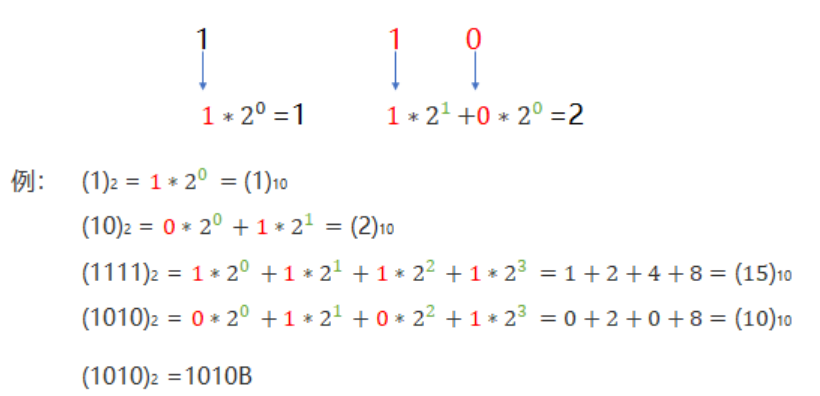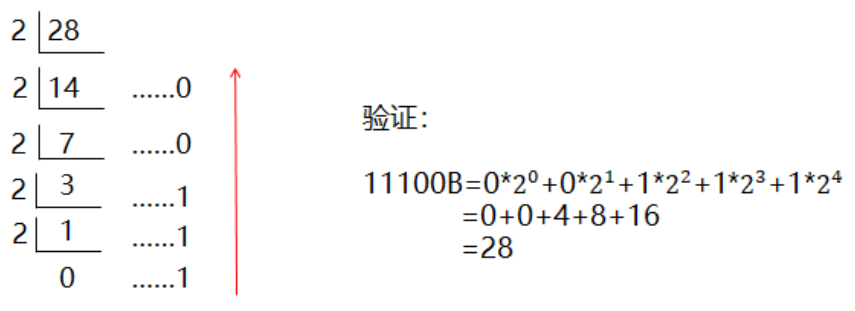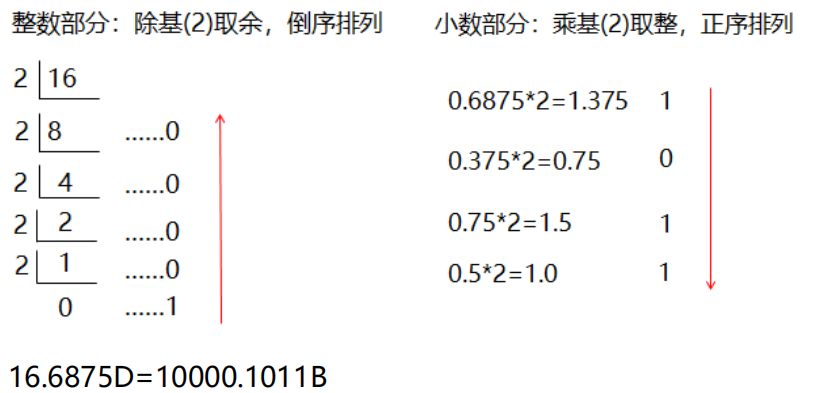### 八进制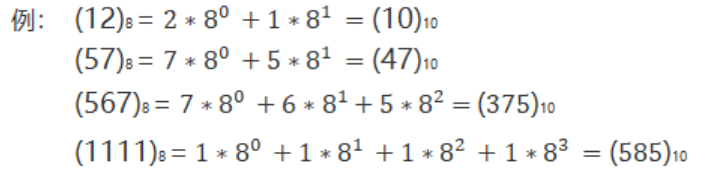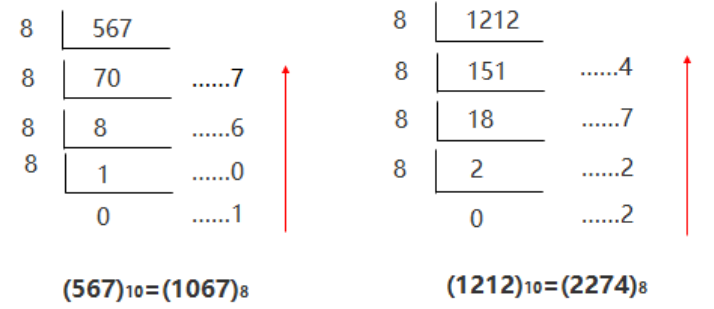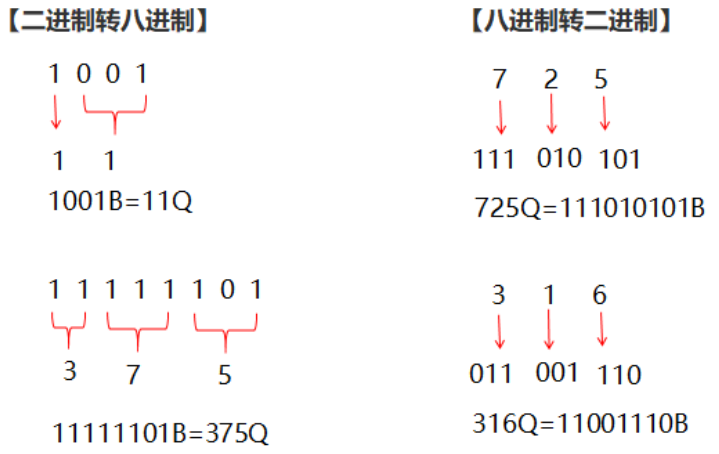### 十六进制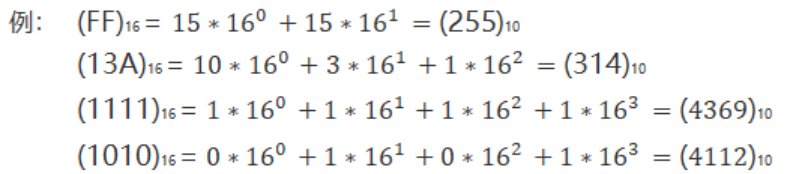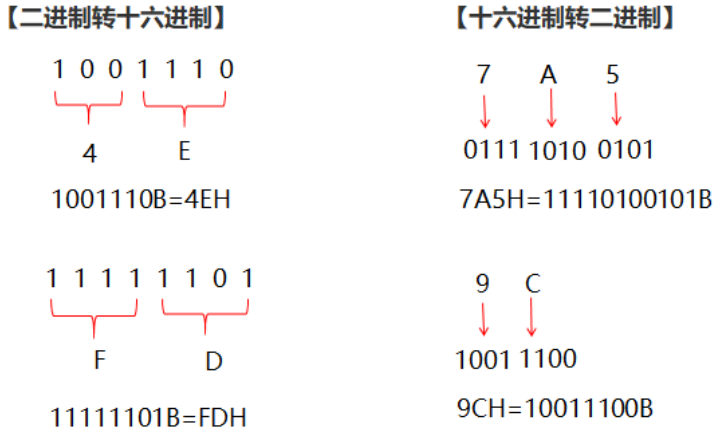``#include<bits/stdc++.h>using namespace std; int fun(int x){    int i=1,sum=0;    while(x!=0)    {        sum=sum+(x%%10)*i;        x=x/10;        i=i*8;    }    return sum;}int main() {    int x;    cin>>x;    cout<<fun(x)<<endl;}``

``#include<bits/stdc++.h>using namespace std; int main() {    int n=0,x,a;    cin>>x;    while(x!=0)    {        n++;        a[n]=x%%8;        x=x/8;    }    for(int i=n;i>=1;i--)         cout<<a[i];    return 0; }``

101 2

样例输出1：

5

解题思路：将数字存在字符串里，从后往前遍历整个字符串，因为可能是16进制会有ABCDEF，因此需要对a[i]进行判断，如果是a[i]>='A'则t=a[i]-65+10，否则t=a[i]-48。然后将t乘上b的k次方就可以了，k从0开始每次加1。

``#include<bits/stdc++.h>using namespace std;int main( ){    string a;        //将数字存在字符串里    int b,i,s=0,k=0,t;    cin>>a>>b;       //输入这个数和进制    for(i=a.size()-1;i>=0;i--)  //从后往前    {        t=(a[i]>='A'?a[i]-65+10:a[i]-48); //因为可能是16进制会有ABCDEF，因此需要对a[i]进行判断，如果是a[i]>='A'则t=a[i]-65+10，否则t=a[i]-48。        s=s+t*pow(b,k); //pow函数的用法，表示b的k次方        k++; //k+1，你懂的    }    cout<<s; }   ``

101 8

样例输出1：

145

``#include<bits/stdc++.h>using namespace std;int main( ){    int a,b;    string c;  //c是字符串    cin>>a>>b;    while(a!=0)    {        char t=a%%b+48;  //t是字符型，把数字存进字符串里加48        if(t>'9') //这里是字符’9’，考虑16进制        {            t=64+t-'9';  //如果超过9，转换成字母ABCDEF        }         c=c+t; //字符串拼接        a=a/b; //更新a的值    }    reverse(c.begin(),c.end()); //逆序输出即可    cout<<c;}   ``

101 10 8

145

``#include<bits/stdc++.h>using namespace std;int _zhuan10(string a,int b){    int i,s=0,k=0,t;    for(i=a.size()-1;i>=0;i--)    {        t=(a[i]>='A'?a[i]-65+10:a[i]-48);        s=s+t*pow(b,k);        k++;    }    return s;}string _10zhuan(int a,int b){    string c;    while(a!=0)    {        char t=a%%b+48;        if(t>'9')        {            t=64+t-'9';         }         c=c+t;        a=a/b;    }    reverse(c.begin(),c.end());    return c;   } int main( ){    string a;    int b,c;    cin>>a>>b>>c;    int t=_zhuan10(a,b);    cout<<_10zhuan(t,c); }   ``